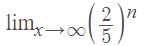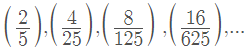# Find the Limit of a Sequence: Intro / Simple Steps

A sequence is a list of ordered numbers like (1, 2, 3, …) or (0.9, 0.999. 0.99999). The limit of a sequence is the limit of a list of discrete numbers: what the list “tends” towards as the number of terms gets bigger and bigger.

For example, the limit of the sequence (0.9, 0.09, 0.009, 0.0009…) converges to zero. On the other hand, the limit of the sequence (1, 2, 3, 4, 5…) is divergent: it’s never going to settle down and will always get larger and larger.

The limit of a sequence is similar to the idea of limits for functions, so to find the limit of a sequence, we usually take a look at the generating function to see what happens.

## Find the Limit of a Sequence: Tips and Examples

Tip #1: Calculate a few terms to see what happens.

• Example #1:The first few terms are:These fractions are getting smaller and smaller, so the limit converges to 0.

• Example #2:Calculating the first few terms, starting with n = 1, gives us:
(2, 4, 8, 16, …).

The numbers are getting larger and larger; The limit diverges → ∞.

• Example #3:The first few terms are:These fractions are getting smaller and smaller, so the limit converges to zero.

Tip #2: Divide by the largest degree of n..

This is ideal for more challenging sequences with terms like n2 or n3. This is a classic method, cropping up in many textbooks. However, it does require some strong algebra skills.

Example #4:Dividing by the highest degree n (which is n2 in this example), and simplifying, this expression becomes:Next, notice the two fractions to the right in the numerator and denominator? Those will tend to zero as n gets large (as in example #3), so we can ignore them. That means our limit will converge to 4/7.

CITE THIS AS:
Stephanie Glen. "Find the Limit of a Sequence: Intro / Simple Steps" From StatisticsHowTo.com: Elementary Statistics for the rest of us! https://www.statisticshowto.com/find-the-limit-of-a-sequence-example/